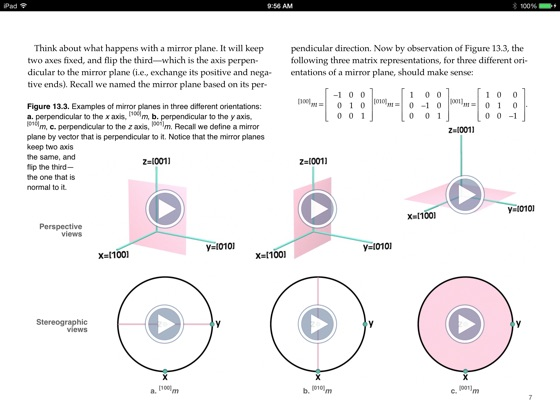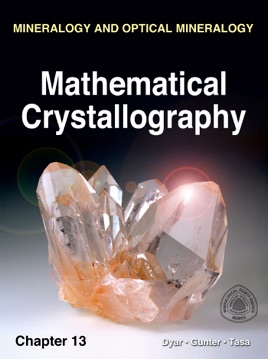• \$3.99

## Publisher Description

Mathematical crystallography was developed to provide a unifying method for understanding crystal symmetry based on matrix algebra. The external symmetry of mineral crystals is often controlled by the internal symmetry of the atomic arrangement. The mathematics behind this internal symmetry is examined and explained in great detail.

Topics covered in Chapter 13 include:

Matrix Representation of Symmetry Operations
Rotoinversion Axes
Derivation of Space Group Symmetry Operations
The Metric Tensor
Volume Calculations
Bond Distances
BondAngles
d-spacings and Reciprocal Space
Angles Between Two Axes
Angles Between Two Planes
Angles Between an Axis and a Plane Normal
Derivation of the 32 Crystallographic 3-D Point Groups
Proper Point Groups
Improper Point Groups
Grouping the 32 Point Groups into the Six Crystal Systems
A Brief Introduction to Linear Algebra and Matrix Manipulation as Applied to Mineralogy

Multiplication of Matrices
Transpose of a Matrix
Inversion ("Division") of Matrices
The Determinate of a Matrix
Addition and Subtraction of Matrices
Dot Products
Cross Products

The General Cartesian Rotation Matrix and Its Use in Mineralogy

•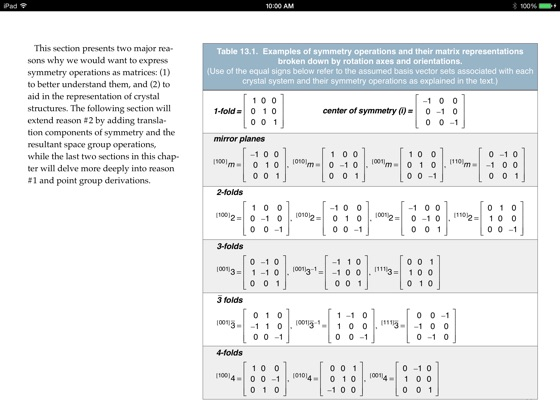••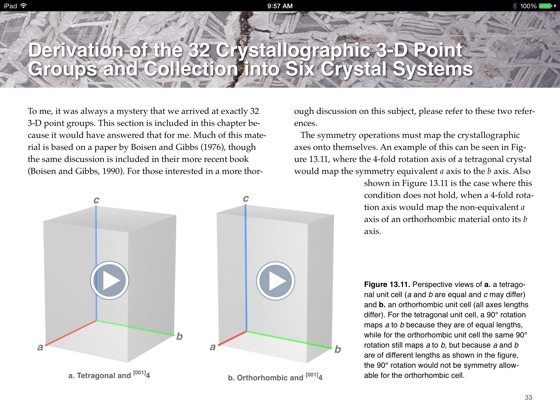•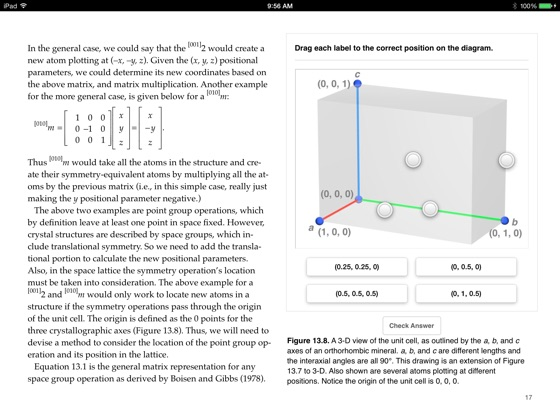•